## CONLANG@LISTSERV.BROWN.EDU

#### View:

 Message:[First|Previous|Next|Last] By Topic:[First|Previous|Next|Last] By Author:[First|Previous|Next|Last] Font: Proportional Font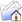LISTSERV Archives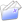CONLANG HomeCONLANG September 1998, Week 4

Subject:Re: equinox

From:Date:Sat, 26 Sep 1998 20:35:03 GMT

Content-Type:text/plain

Parts/Attachments: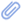text/plain (28 lines)
 ```JOEL MATTHEW PEARSON <[log in to unmask]> wrote: >=20 > On Fri, 25 Sep 1998, Nik Taylor wrote: > > The books are called Winter on _______ and Summer on _______ and > > Spring on ________, where the blank is whatever the heck the planet = is > > called. I don't remember. >=20 > Ooh, ooh! The author is Brian Aldiss. I don't remember the name of > the planet, though.      Helliconia. =3D=3D=3D=3D=3D=3D=3D=3D=3D=3D=3D=3D=3D=3D=3D=3D=3D=3D=3D=3D=3D=3D=3D=3D=3D= =3D=3D=3D=3D=3D=3D=3D=3D=3D=3D=3D=3D=3D=3D=3D=3D=3D=3D=3D=3D=3D=3D=3D=3D=3D= =3D=3D=3D=3D=3D=3D=3D=3D=3D=3D=3D=3D=3D=3D=3D=3D=3D=3D=3D=3D=3D=3D=3D=3D=3D= =3D              Dennis Paul Himes <> [log in to unmask]              homepage: http://www.connix.com/~dennis/dennis.htm         Gladilatian page: http://www.connix.com/~dennis/glad/lang.htm =20 Disclaimer: "True, I talk of dreams; which are the children of an idle brain, begot of nothing but vain fantasy; which is as thin of substance = as the air." - Romeo & Juliet, Act I Scene iv Verse = 96-99 ```

#### Search Archives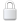Log In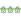Get Password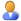Subscribe or Unsubscribe